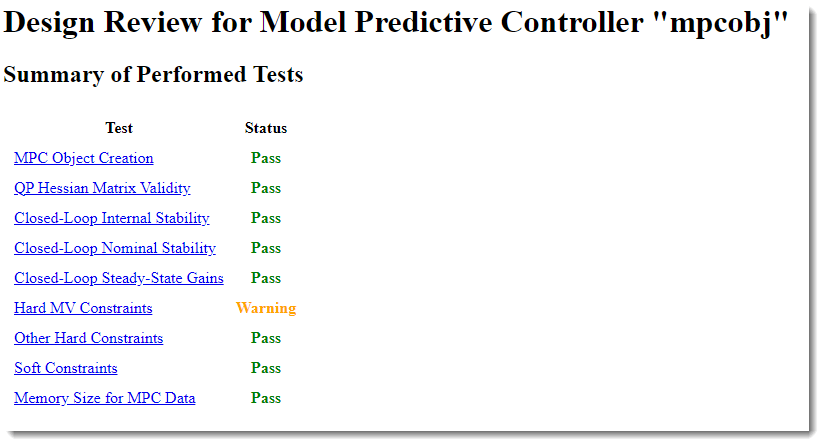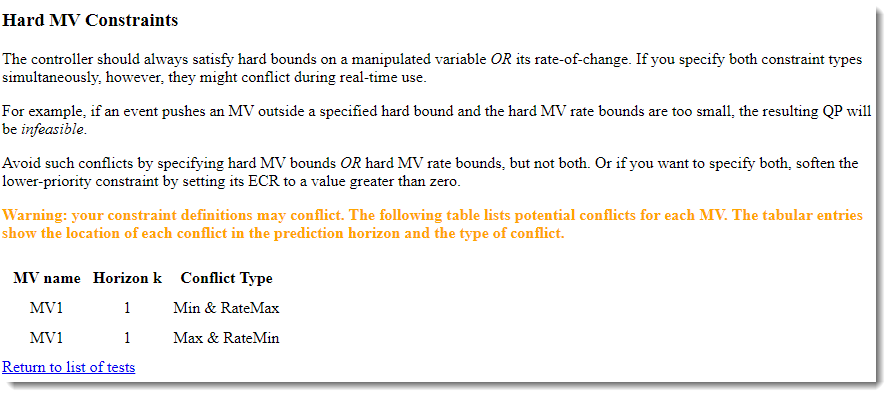# review

Examine MPC controller for design errors and stability problems at run time

## Syntax

``review(mpcobj)``
``results = review(mpcobj)``

## Description

example

````review(mpcobj)` checks for potential design issues in the model predictive controller with defined sample time, `mpcobj`, and generates a testing report. The testing report provides information about each test, highlights test warnings and failures, and suggests possible solutions. For more information on the tests performed by the `review` function, see Algorithms.```

example

````results = review(mpcobj)` returns the test results and suppresses the testing report. ```

## Examples

collapse all

Define a plant model, and create an MPC controller.

```plant = tf(1,[10 1]); mpcobj = mpc(plant,2);```
```-->"PredictionHorizon" is empty. Assuming default 10. -->"ControlHorizon" is empty. Assuming default 2. -->"Weights.ManipulatedVariables" is empty. Assuming default 0.00000. -->"Weights.ManipulatedVariablesRate" is empty. Assuming default 0.10000. -->"Weights.OutputVariables" is empty. Assuming default 1.00000. ```

Set hard upper and lower bounds on the manipulated variable and its rate of change.

```MV = mpcobj.MV; MV.Min = -2; MV.Max = 2; MV.RateMin = -4; MV.RateMax = 4; mpcobj.MV = MV;```

Review the controller design. The review function generates and opens a report in the Web Browser window.

`review(mpcobj)`
```-->Converting the "Model.Plant" property to state-space. -->Converting model to discrete time. -->Assuming output disturbance added to measured output #1 is integrated white noise. -->"Model.Noise" is empty. Assuming white noise on each measured output. Test report has been saved to: /tmp/Bdoc23b_2361005_3589793/index.html ````review` flags a potential constraint conflict that could result if this controller was used to control a real process. To view details about the warning, click Hard MV Constraints.Define a plant model with no direct feedthrough.

```plant = rss(3,1,1); plant.D = 0; Ts = 0.1;```

Create an MPC controller.

`mpcobj = mpc(plant,Ts);`
```-->"PredictionHorizon" is empty. Assuming default 10. -->"ControlHorizon" is empty. Assuming default 2. -->"Weights.ManipulatedVariables" is empty. Assuming default 0.00000. -->"Weights.ManipulatedVariablesRate" is empty. Assuming default 0.10000. -->"Weights.OutputVariables" is empty. Assuming default 1.00000. ```

Specify constraints for the controller.

```MV = mpcobj.MV; MV.Min = -2; MV.Max = 2; MV.RateMin = -4; MV.RateMax = 4; mpcobj.MV = MV;```

Review the controller design, and suppress the testing report.

`results = review(mpcobj)`
```-->Converting model to discrete time. -->Assuming output disturbance added to measured output #1 is integrated white noise. -->"Model.Noise" is empty. Assuming white noise on each measured output. ```
```results = struct with fields: ObjectCreation: Pass HessianMatrix: Pass InternalStability: Pass NominalStability: Pass SteadyState: Pass HardMVConstraints: Warning HardOtherConstraints: Pass SoftConstraints: Pass ```

All of the tests passed, except for the hard MV constraints test, which generated a warning.

Create and review designs for gain-scheduled model predictive controllers for two plant operating conditions.

Define the model parameters.

```M1 = 1; M2 = 5; k1 = 1; k2 = 0.1; b1 = 0.3; b2 = 0.8; yeq1 = 10; yeq2 = -10;```

Create plant models for each of the two operating conditions.

```A1 = [0 1; -k1/M1 -b1/M1]; B1 = [0 0; -1/M1 k1*yeq1/M1]; C1 = [1 0]; D1 = [0 0]; sys1 = ss(A1,B1,C1,D1); sys1 = setmpcsignals(sys1,'MV',1,'MD',2); A2 = [0 1; -(k1+k2)/(M1+M2) -(b1+b2)/(M1+M2)]; B2 = [0 0; -1/(M1+M2) (k1*yeq1+k2*yeq2)/(M1+M2)]; C2 = [1 0]; D2 = [0 0]; sys2 = ss(A2,B2,C2,D2); sys2 = setmpcsignals(sys2,'MV',1,'MD',2);```

Design an MPC controller for each operating condition.

```Ts = 0.2; p = 6; m = 2; MPC1 = mpc(sys1,Ts,p,m);```
```-->"Weights.ManipulatedVariables" is empty. Assuming default 0.00000. -->"Weights.ManipulatedVariablesRate" is empty. Assuming default 0.10000. -->"Weights.OutputVariables" is empty. Assuming default 1.00000. ```
`MPC2 = mpc(sys2,Ts,p,m);`
```-->"Weights.ManipulatedVariables" is empty. Assuming default 0.00000. -->"Weights.ManipulatedVariablesRate" is empty. Assuming default 0.10000. -->"Weights.OutputVariables" is empty. Assuming default 1.00000. ```
`controllers = {MPC1,MPC2};`

Review the controller designs, and store the test result structures.

```for i = 1:2 results(i) = review(controllers{i}); end```
```-->Converting model to discrete time. -->Assuming output disturbance added to measured output #1 is integrated white noise. -->"Model.Noise" is empty. Assuming white noise on each measured output. -->Converting model to discrete time. -->Assuming output disturbance added to measured output #1 is integrated white noise. -->"Model.Noise" is empty. Assuming white noise on each measured output. ```

## Input Arguments

collapse all

MPC controller object, specified as an `mpc` object.

## Output Arguments

collapse all

Test results, returned as a structure with the following fields:

• `ObjectCreation` — MPC object creation test

• `HessianMatrix` — QP Hessian matrix validity test

• `InternalStability` — Internal stability test

• `NominalStability` — Nominal stability test

• `SteadyState` — Closed-loop steady-state gains test

• `HardMVConstraints` — Hard MV constraints test

• `HardOtherConstraints` — Other hard constraints test

• `SoftConstraints` — Soft constraints test

For more information on the tests performed by the `review` function, see Algorithms.

The `results` structure does not contain a field for the Memory Size for MPC Data test.

For each test, the result is returned as one of the following:

• `1` — Pass

• `0` — Warning

• `-1` — Fail

If a given test generates a warning or fails, generate a testing report by calling `review` without an output argument. The testing report provides details about the warnings and failures, and suggests possible solutions.

## Tips

• You can also review your controller design in the MPC Designer app. On the Tuning tab, in the Analysis section, click Review Design.

• Test your controller design using techniques such as simulations, since `review` cannot detect all possible performance factors.

## Algorithms

The review command performs the following tests.

TestDescription
MPC Object CreationTests whether the controller specifications generate a valid MPC controller. If the controller is invalid, additional tests are not performed.
QP Hessian Matrix ValidityTests whether the MPC quadratic programming (QP) problem for the controller has a unique solution. You must choose cost function parameters (penalty weights) and horizons such that the QP Hessian matrix is positive-definite.
Closed-Loop Internal StabilityExtracts the `A` matrix from the state-space realization of the unconstrained controller, and then calculate its eigenvalues. If the absolute value of each eigenvalue is less than or equal to `1` and the plant is stable, then your feedback system is internally stable.
Closed-Loop Nominal StabilityExtracts the `A` matrix from the discrete-time state-space realization of the closed-loop system; that is, the plant and controller connected in a feedback configuration. Then calculate the eigenvalues of `A`. If the absolute value of each eigenvalue is less than or equal to `1`, then the nominal (unconstrained) system is stable. This test is not performed if `mpcobj` is set to use a custom state estimator.
Closed-Loop Steady-State GainsTests whether the controller forces all controlled output variables to their targets at steady state in the absence of constraints. This test is not performed if `mpcobj` is set to use a custom state estimator. This test is not performed if `mpcobj` is set to use a custom state estimator.
Hard MV ConstraintsTests whether the controller has hard constraints on both a manipulated variable and its rate of change, and if so, whether these constraints may conflict at run time.
Other Hard ConstraintsTests whether the controller has hard output constraints or hard mixed input/output constraints, and if so, whether these constraints may become impossible to satisfy at run time.
Soft ConstraintsTests whether the controller has the proper balance of hard and soft constraints by evaluating the constraint ECR parameters.
Memory Size for MPC DataEstimates the memory size required by the controller at run time.

## Alternatives

`review` automates certain tests that you can perform at the command line.

## Version History

Introduced in R2011b